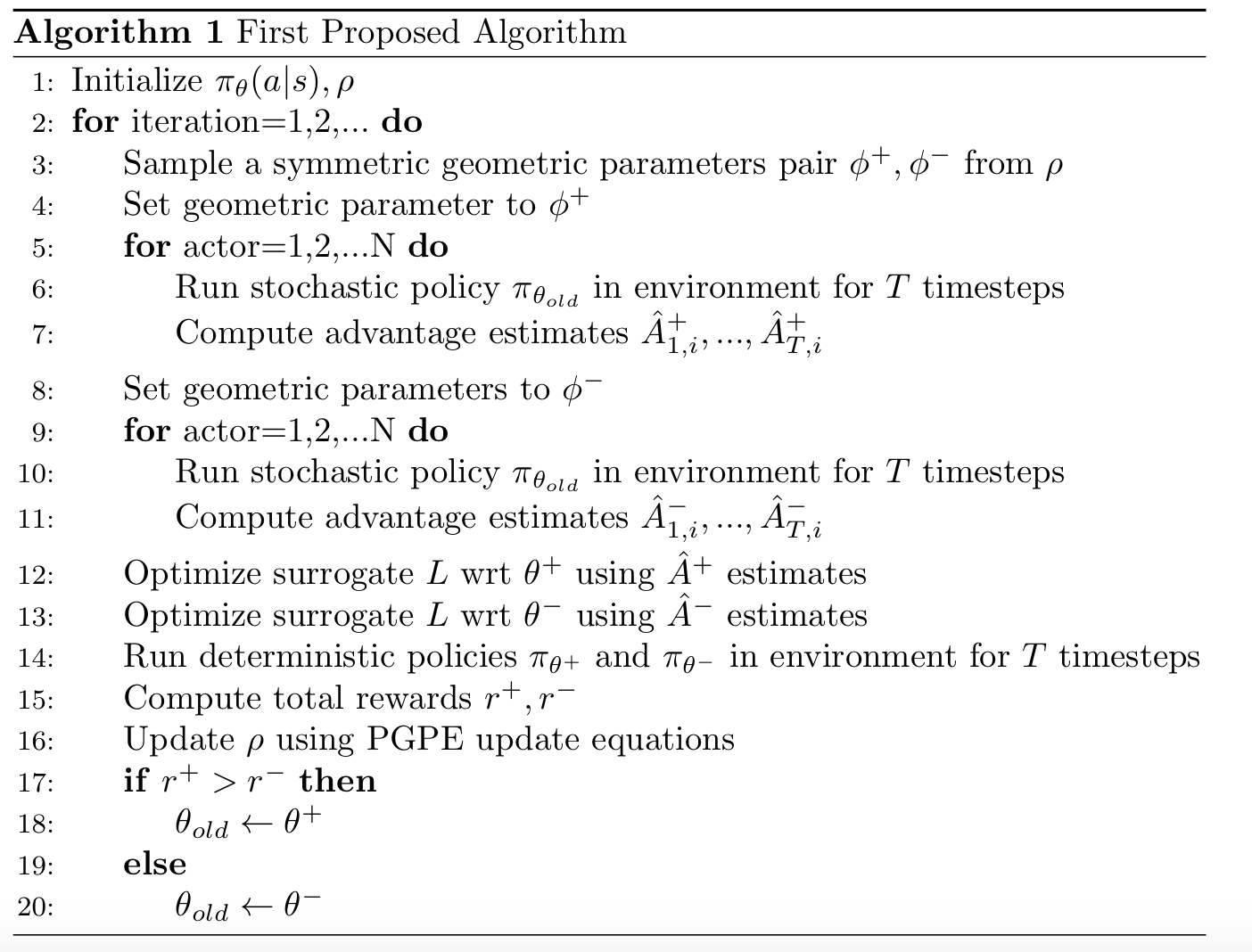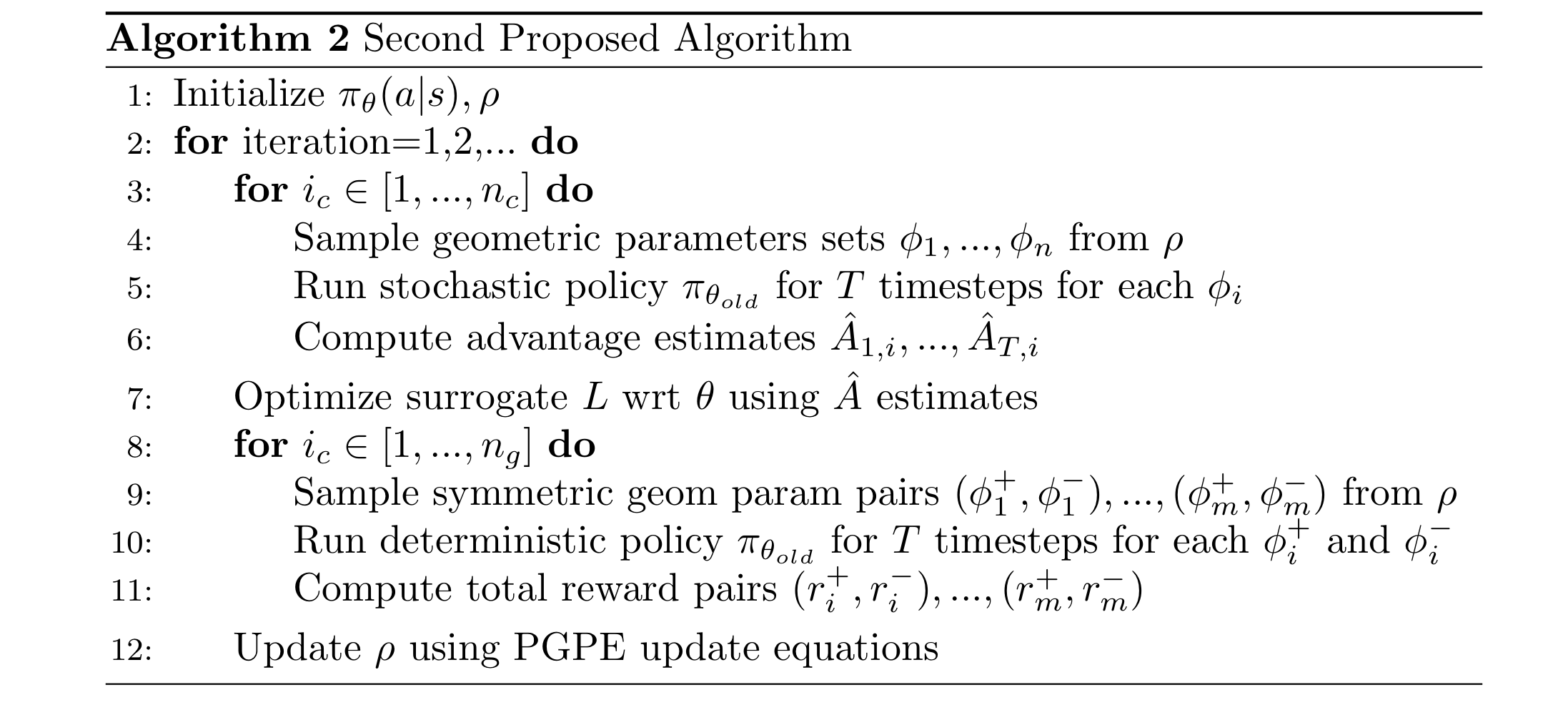26 Feb

#### Comparison of Co-Optimization Algorithms

A recent blog post by Christian outlines an algorithm that we formulated for the co-optimization of structure and control of robots. This algorithm seeks to more tightly couple the co-optimization as compared to the algorithm described in . Let's call the algorithm we propose Algorithm 1.Algorithm 1 samples two robot designs that are symmetric around the center of the normal distribution they are sampled from. Then, two separate controllers are optimized for each of these symmetric robot design samples and then the performances of the two designs using updated controllers are measured. These measurements are used to update the parameters of the design distribution as well as choose which controller to continue with.

We also propose a second algorithm (Algorithm 2) that is mainly the same algorithm that is proposed in  but with a slight but potentially important change.does not seem to mention whether the policy that is used for the geometric parameter optimization is deterministic or stochastic. Christian and I hypothesize that using a deterministic policy for the geometric parameter optimization will result in a less noisy gradient for updating the parameter distribution. This is the main difference between this algorithm and the one in . In addition, the paper does not indicate whether the PGPE they used is SyS-MultiPGPE or just MultiPGPE without symmetric sampling. Our algorithm explicitly uses SyS-MultiPGPE.

The main difference between Algorithm 1 and Algorithm 2 is that 1 will attempt to optimize the controller specifically for certain geometries which could lead to it discovering controller-geometry pairs that are more specifically suited to one another. In comparison, Algorithm 2 will find a general controller that works well and will search in the geometric parameter space using that controller. This can be interpreted as Algorithm 1 more tightly coupling geometry and control as it will take one step in controller parameter space and then take the next step in geometric parameter space. 2 takes multiple steps in controller space followed by multiple steps in geometry space which could lead to it missing some optima that may be better.

 Charles Schaff, David Yunis, Ayan Chakrabarti, Matthew R. Walter. Jointly Learning to Construct and Control Agents using Deep Reinforcement Learning. arXiv:1801.01432, 2018.